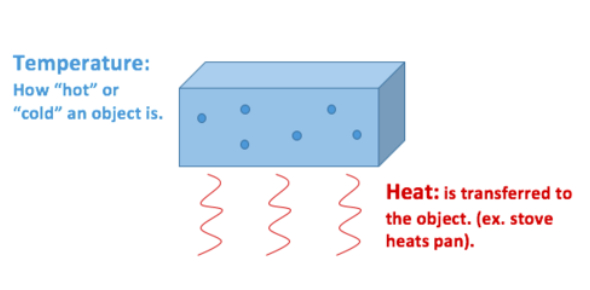# Physics Quiz On Heat And Temperature!

10 Questions | Attempts: 2749SettingsHeat and Temperature are interlinked but both are distinct concepts. When you heat anything, temperature rises or lower down according to the environment. Heat is the absolute energy while the temperature is the measurement. This quiz has been created to test and enhance your knowledge about Heat and Temperature. So, let's try out the quiz. All the best!

• 1.
When a material is heated:
• A.

The molecules lose kinetic energy

• B.

The molecules in the substance will be grouped more closely

• C.

The internal energy can be calculated from the change in temperature

• D.

The total internal energy of the substance will be increased

• 2.
A hot water tap delivers water at 80 degree Celcius. Ten litres of hot water is added to a pail containing 30 litres of water at 20 degree Celcius. Ignoring energy losses to the surrounding environment, what will be the final temperature of the water int he pail?
• A.

25 degree Celcius

• B.

30 degree Celcius

• C.

35 degree Celcius

• D.

40 degree Celcius

• 3.
50 grams of iron is heated over a flame for several minutes. It is then plunged into a closed container containing 1.0 litre of cold water, originally at 15 degree Celcius. After the temperatures equalize, the water is now found to be at 17 degree Celcius. If no water changed state to become steam and there were no losses to the surrounding environment, what was the temperature of the iron just prior to being immersed in the water?
• A.

399 degree Celcius

• B.

400 degree Celcius

• C.

401 degree Celcius

• D.

402 degree Celcius

• 4.
A balloon is filled with helium gas to a volume of 30 litres at 1.0 atm pressure. The balloon is released and rises to an altitude where its volume expands to 180 litres. Calculate the pressure being exerted on the balloon at thins altitude.
• A.

0.15 atm

• B.

0.16 atm

• C.

0.17 atm

• D.

0.18 atm

• 5.
A fixed volume of gas has a pressure of 0.85 atm at 20 degree Celcius. The gas is heated to 85 degree Celcius. What is the new pressure?
• A.

0.5 atm

• B.

1.0 atm

• C.

1.5 atm

• D.

2.0 atm

• 6.
A sample of air at room temperature has a volume of 90.0 mL at 95.0 kPa pressure. If the air remains at room temperature, what volume will it occupy if the pressure is changed to 40.0 kPa?
• A.

212.00 mL

• B.

212.75 mL

• C.

213.50 mL

• D.

213.75 mL

• 7.
At 1 atm pressure and 25 degree Celcius, a gas-filled balloon has a volume of 20.0 L. The balloon is cooled down to -5 degree Celcius. What would be the volume of the balloon now, assuming pressure remains constant?
• A.

17.00 L

• B.

17.49 L

• C.

17.79 L

• D.

17.99 L

• 8.
What is it called when heat transfers from one object to another?
• A.

Induction

• B.

Expansion

• C.

Equilibrium

• D.

Deduction

• E.

Conduction

• 9.
What happens to the molecules of a substance as it gets cooler?
• A.

The molecules shrink

• B.

The molecules expand

• C.

The molecules move faster

• D.

The molecules move slower

• E.

Nothing

• 10.
Which of the following is responsible for bringing heat to our planet?
• A.

Convection

• B.

Conduction

• C.Back to top